ExampleC.mws

 > with(linalg):

 > #I show a trade secret of how to produce complicated looking matrices having a nice Jordan form

 > A:=matrix([[3, 1, 0, 0, 0, 0, 0], [0, 3, 1, 0, 0, 0,0], [0, 0, 3, 0, 0, 0, 0], [0, 0, 0, 3,1, 0, 0], [0, 0, 0, 0, 3, 0, 0], [0, 0, 0, 0, 0, 2, 1], [0, 0, 0, 0, 0, 0, 2]]);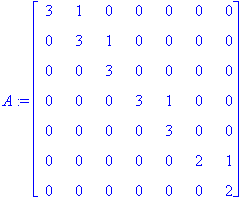> M:=matrix([[1, 0, 3, 0, 3, 0, 3], [0,1, 1, 1, 1, 1, 1], [0, 0, 1, 0, 3, 0, 3], [0, 0, 0, 1, 1, 1, 1], [0, 0, 0, 0, 1, 1, 1], [0, 0, 0, 0, 0, 1, 3], [0, 0, 0, 0, 0, 0, 1]]);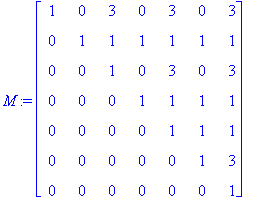> #The fact that M has determinant 1 guarantees it's invertible over Z and so that the overall scrambling we are doing is not too bad numerically

 > S:=matrix([[0, 1, 0, 0, 0, 0, 0], [0, 0, 1, 0, 0, 0, 0], [0, 0, 0, 1, 0, 0, 0], [0, 0, 0, 0, 1, 0, 0], [0, 0, 0, 0, 0, 1, 0], [0, 0, 0, 0, 0, 0, 1], [1, 0, 0, 0, 0, 0, 0]]);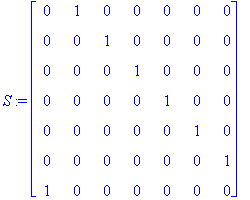> #S is just a permuation matrix designed to kill the upper diagonal form of the rest of the matrices. And here comes the matrix we'll deal with:

 > B:=multiply(M, inverse(S), A, S, inverse(M));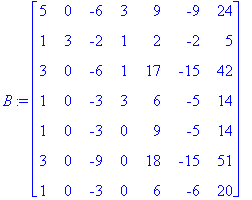> factor(charpoly(B, t));factor(minpoly(B, t));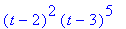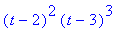> #From this we know we deal with two spaces. Note that we know that there is one Jordan block corresponding to the eigenvalue 2 and it has size 2, however we don't know yet the basis in which we get that block. The situation for 3 is worse. We only know there's one Jordan block of size 3 and the total size is 5. We could have a priori either a (3, 2) situation or a (3, 1, 1) situation. We first deal with the space associated with the factor (t-3)^5.

 > L1:=kernel(B-3);nops(L1);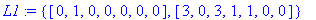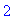> L2:=kernel((B-3)^2);nops(L2);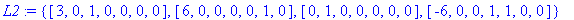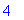> L3:=kernel((B-3)^3);nops(L3);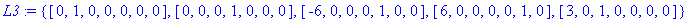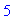> #We have calculated the dimensions of the kernels and bases. In our notation from class we should have C^3 one dimensional (=5-4), C^2 is two dimensionl(=4-2) and contains (B-3)C^3 and C^1 is 2 dimensional (=dim kernel B-3) and contains (B-3)C^2. We span C^3 using a vector in kernel (B-3)^3 and not in kernel (B_3)^2. We try:

 > multiply((B-3)^2, L3);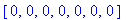> multiply((B-3)^2, L3);> multiply((B-3)^2, L3);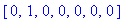> multiply((B-3)^2, L3);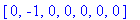> multiply((B-3)^2, L3);> v3:=vector([-6, 0, 0, 0, 1, 0, 0]);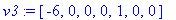> #(Hopefully, when you run Maple again on this example it will indeed choose to include this vector in the basis L3....)

 > #Then (B-3)v3 is a vector in kernel (B-3)^2. It is

 > Uv3:=multiply(B-3, v3);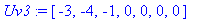> #We are looking for one more vector v2 so that kernel (B-3)^2 is the direct sum of kernel(B-3) and Span((B-3)v3, v2). Namely, for a vector in kernel (B-3)^2 and not in the span of the union of L1 and (B-3)v3. We can find such a vector among L2. We are looking for a vector in L2 so that the matrix below has rank 4.

 > matrix([L1, L1, Uv3, L2]);rank(%);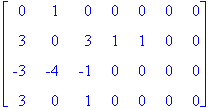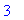> matrix([L1, L1, Uv3, L2]);rank(%);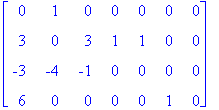> matrix([L1, L1, Uv3, L2]);rank(%);> matrix([L1, L1, Uv3, L2]);rank(%);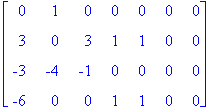> #So we may take (again, provided Maple chooses the list L2 in the same way when you try it out...)

 > v2:=vector([6, 0, 0, 0, 0, 1, 0]);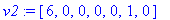> #At this point we are done with our basis for the t-3 factor. It is

 > #  U2v3= (B-3)^2v3,   Uv3=(B-3)v3,    v3,    Uv2=(B-3)v2,   v2,

 > #where

 > U2v3:=multiply((B-3)^2, v3);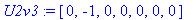> Uv2:=multiply((B-3), v2);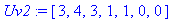> #The t-2 factor is easier

 > H1:=kernel((B-2)^2);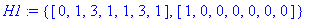> H2:=kernel(B-2);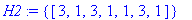> #We look for a vector in H1 and not in H2. For example, we can take

 > w2:=vector([1, 0, 0, 0, 0, 0, 0]);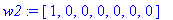> Uw2:=multiply(B-2, w2);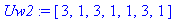> #Our basis is then the columns of the matrix

 > M:=transpose(matrix([U2v3, Uv3, v3, Uv2, v2, Uw2, w2]));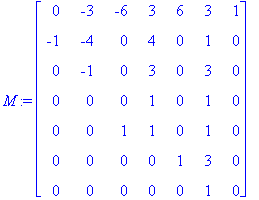> multiply(inverse(M), B, M);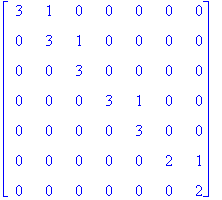> #DONE!!